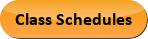Sep 21, 2023
2022-2023 College Catalog
 Select a Catalog 2023-2024 College Catalog 2022-2023 College Catalog [ARCHIVED CATALOG] 2021-2022 College Catalog [ARCHIVED CATALOG] 2020-2021 College Catalog [ARCHIVED CATALOG] 2019-2020 College Catalog [ARCHIVED CATALOG] *** DRAFT 2024-2025 College Catalog DRAFT *** [ARCHIVED CATALOG]
 HELP 2022-2023 College Catalog [ARCHIVED CATALOG] Print-Friendly Page (opens a new window) Add to Portfolio (opens a new window)

# MAT 172 - Finite Mathematics

3 Credits, 3 Contact Hours
3 lecture periods 0 lab periods

Sampling of finite mathematics which includes mathematics of finance, linear business functions, systems of equations, matrices, geometric and simplex methods of solving linear programming problems, logic, sets, combinatorics, basic probability, probability distributions, and Markov chains.

Prerequisite(s): Within the last three years: MAT 151  with a grade of C or better or satisfactory score on the mathematics assessment exam.
Gen-Ed: Meets AGEC - MATH; Meets CTE - M&S.Course Learning Outcomes
1. Solve linear programing problems.
2. Calculate and apply probabilities.
3. Solve problems involving real world applications.

Performance Objectives:
1. Apply the mathematics of finance.
2. Graph and evaluate linear functions, including those applying to business.
3. Solve systems of linear equations using algebraic methods.
4. Perform algebraic operations with matrices.
5. Solve systems of linear equations using matrices; use Gaussian elimination with augmented matrices; use inverse matrices.
6. Solve linear programming problems geometrically.
7. Solve linear programming problems using the simplex method.
8. Apply basic symbolic logic including truth tables.
9. Represent sets using Venn Diagrams and perform set operations.
10. Use the principles of counting (including the multiplication principle, combinations, and permutations) to solve counting problems.
11. Calculate probabilities using conditional probability, independence, and Bayes’ theorem.
12. Define random variables, differentiating between continuous and discrete; identify probability distributions.

Optional

13. Apply Markov chains, finding transition and distribution matrices.

Outline:
1. Mathematics of Finance
1. Simple interest
2. Compound interest
3. Future and present value of an annuity
4. Amortizations
2. Linear Functions
1. Graphs and equations of lines
2. Linear cost, revenue, and profit functions
3. Linear supply and demand functions
3. Solve Systems of Linear Equations
1. Solve two equations with two unknown variables
2. Break-even points
3. Equilibrium points
4. Matrices
1. Equality of matrices
2. Algebraic operations on matrices
3. Find the inverse of a non-singular matrix
4. Use technology
5. Write matrices which represent a system of linear equations
5. Solving Systems of Linear Equations using Matrices
1. Gaussian elimination
2. Solve a system of equations using inverse matrices
3. Describe in detail the solutions of dependent systems
4. Applications of systems of linear equations
5. Use technology
6. Linear Inequalities and Linear Programming
1. Graph systems of linear inequalities in two variables
2. Solve linear programming problems geometrically
3. Applications of linear programming
7. Solve Linear Programming using the Simplex Method
1. Standard maximization problems
2. Standard minimization problems
3. Duality problems
4. Applications of linear programming
8. Logic
1. Propositions and connectives
2. Apply truth tables to compound proposition
9. Sets
1. Define sets including universal, empty, sub, complement, and power
2. Define and perform set operations
3. Draw Venn Diagrams representing sets
10. Counting
1. Number of elements in a set
2. Number of subsets of a set
3. Multiplication principle
4. Inclusion-exclusion principle
5. Permutations
6. Combinations
7. Applications
11. Probability
1. Sample spaces, simple outcomes, compound outcomes
2. Find the probability of an event
3. Draw tree diagrams
4. Conditional probability
5. Independence
6. Bayes’ theorem
7. Applications
12. Random variables
1. Binomial distribution
2. Normal distribution
3. Expected value
13. Markov chains (Optional)
1. Properties of Markov chains
2. Transition and state matrices
3. Regular Markov chains
4. Absorbing Markov chains

Add to Portfolio (opens a new window)